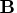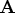Previous Page | Next Page

 Language Reference

## VALSET Call

performs indirect assignment

CALL VALSET( char-scalar, argument);

The inputs to the VALSET subroutine are as follows:
char-scalar
is a character scalar containing the name of a matrix.

argument
is a value to which the matrix is set.
The VALSET subroutine expects a single character string argument containing the name of a matrix. It looks up the matrix and moves the value of the second argument to this matrix. For example, the following statements find that the value of the argumentisand then assign the value 99 to, the indirect result:

```
b="A";
call valset(b,99);
```
The previous value of the indirect result is freed. The following statement setsto 99, but the value ofis unaffected by this statement:
```
b=99;
```Previous Page | Next Page | Top of Page